Скачать презентацию Tentative material to be covered for Exam 2

f9a6d27b69dcd15831f22faf94e3c128.ppt

• Количество слайдов: 25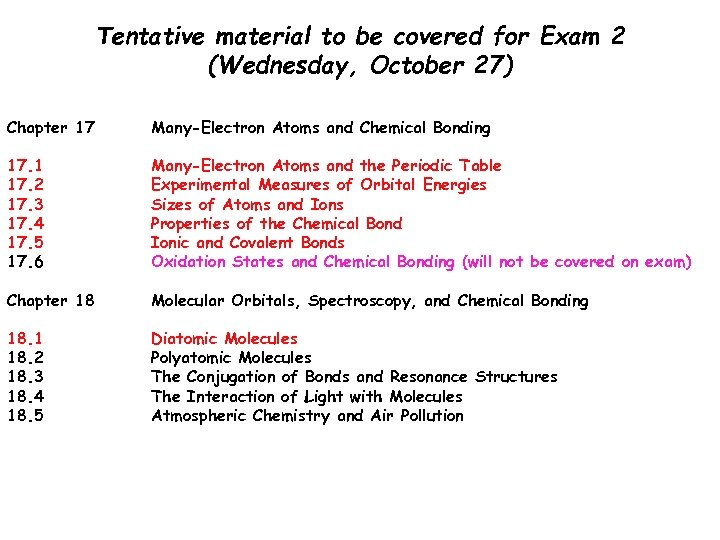Tentative material to be covered for Exam 2 (Wednesday, October 27) Chapter 17 Many-Electron Atoms and Chemical Bonding 17. 1 17. 2 17. 3 17. 4 17. 5 17. 6 Many-Electron Atoms and the Periodic Table Experimental Measures of Orbital Energies Sizes of Atoms and Ions Properties of the Chemical Bond Ionic and Covalent Bonds Oxidation States and Chemical Bonding (will not be covered on exam) Chapter 18 Molecular Orbitals, Spectroscopy, and Chemical Bonding 18. 1 18. 2 18. 3 18. 4 18. 5 Diatomic Molecules Polyatomic Molecules The Conjugation of Bonds and Resonance Structures The Interaction of Light with Molecules Atmospheric Chemistry and Air PollutionNew material on course web site: Practice exams for Chapters 17 and 18 are on line Answers to Chapter 16 will be distributed by tomorrow morning The slide show for Exam 2 has been upgraded. For those having trouble viewing it, please go to room 211 Havemeyer where the slide show is available from 9 -5 A new Powerpoint slide show on material in Chapter 18 has been added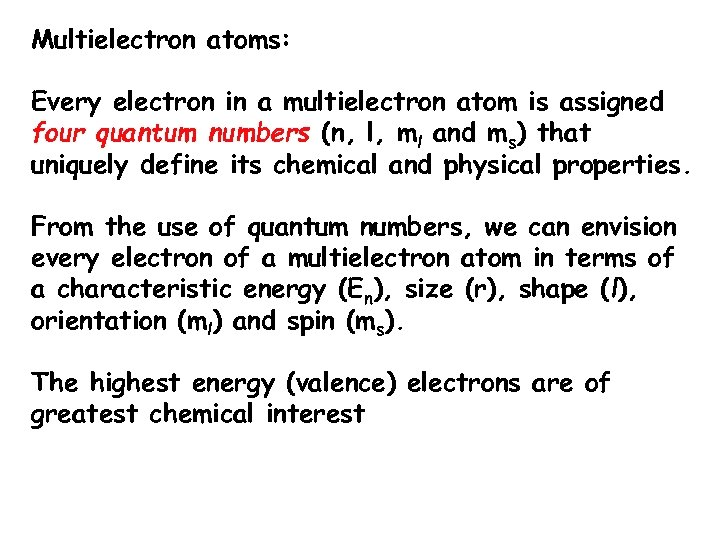Multielectron atoms: Every electron in a multielectron atom is assigned four quantum numbers (n, l, ml and ms) that uniquely define its chemical and physical properties. From the use of quantum numbers, we can envision every electron of a multielectron atom in terms of a characteristic energy (En), size (r), shape (l), orientation (ml) and spin (ms). The highest energy (valence) electrons are of greatest chemical interest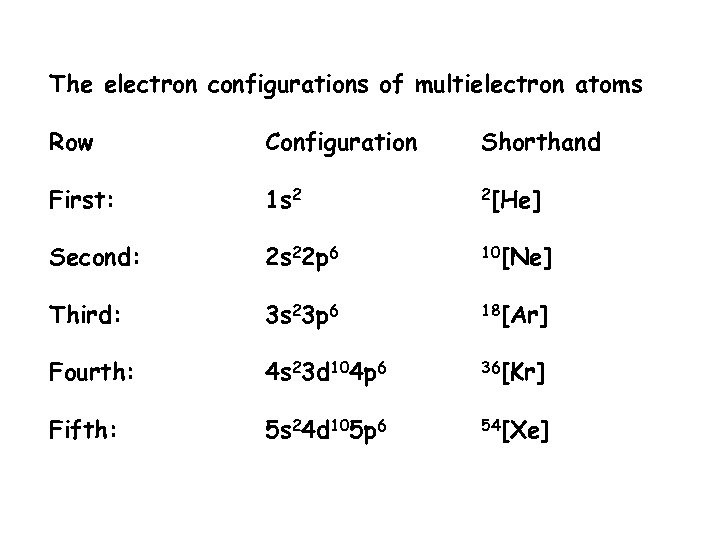The electron configurations of multielectron atoms Row Configuration Shorthand First: 1 s 2 2[He] Second: 2 s 22 p 6 10[Ne] Third: 3 s 23 p 6 18[Ar] Fourth: 4 s 23 d 104 p 6 36[Kr] Fifth: 5 s 24 d 105 p 6 54[Xe]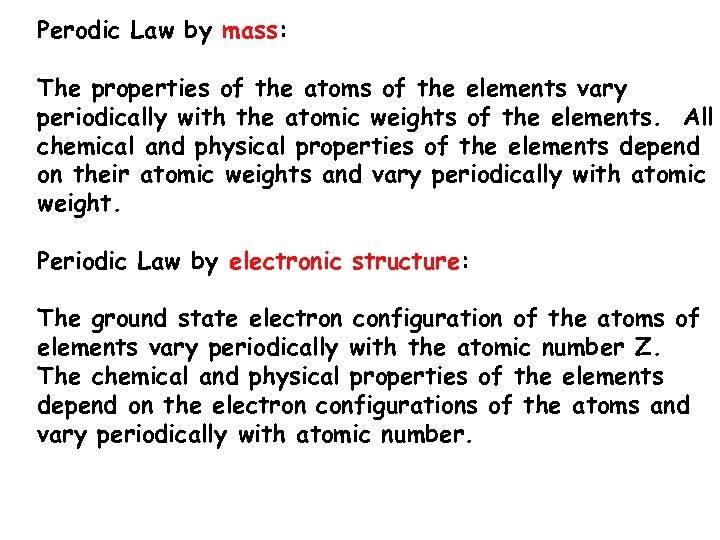Perodic Law by mass: The properties of the atoms of the elements vary periodically with the atomic weights of the elements. All chemical and physical properties of the elements depend on their atomic weights and vary periodically with atomic weight. Periodic Law by electronic structure: The ground state electron configuration of the atoms of elements vary periodically with the atomic number Z. The chemical and physical properties of the elements depend on the electron configurations of the atoms and vary periodically with atomic number.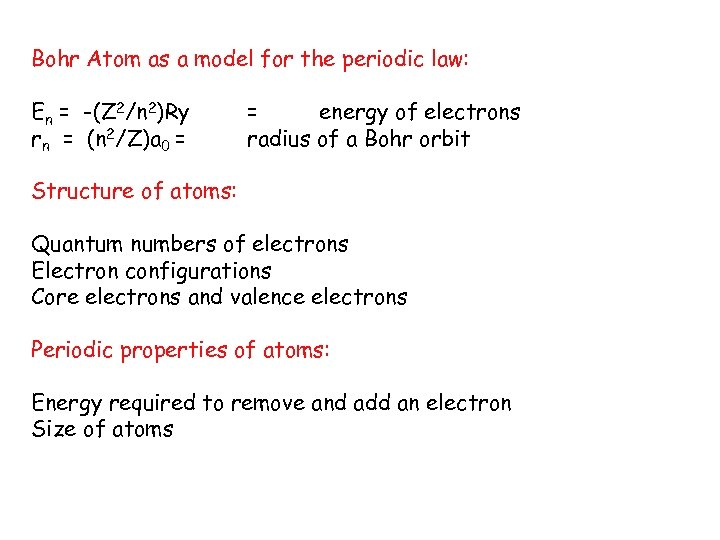Bohr Atom as a model for the periodic law: En = -(Z 2/n 2)Ry rn = (n 2/Z)a 0 = = energy of electrons radius of a Bohr orbit Structure of atoms: Quantum numbers of electrons Electron configurations Core electrons and valence electrons Periodic properties of atoms: Energy required to remove and add an electron Size of atoms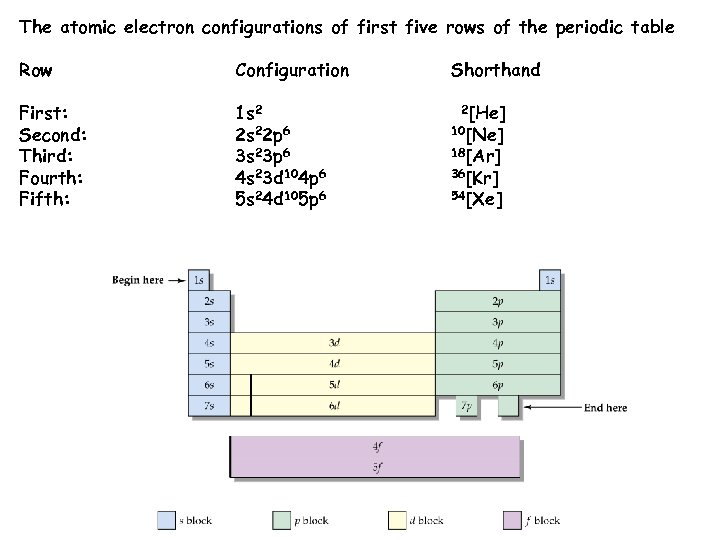The atomic electron configurations of first five rows of the periodic table Row Configuration First: Second: Third: Fourth: Fifth: 1 s 2 2 s 22 p 6 3 s 23 p 6 4 s 23 d 104 p 6 5 s 24 d 105 p 6 Shorthand 2[He] 10[Ne] 18[Ar] 36[Kr] 54[Xe]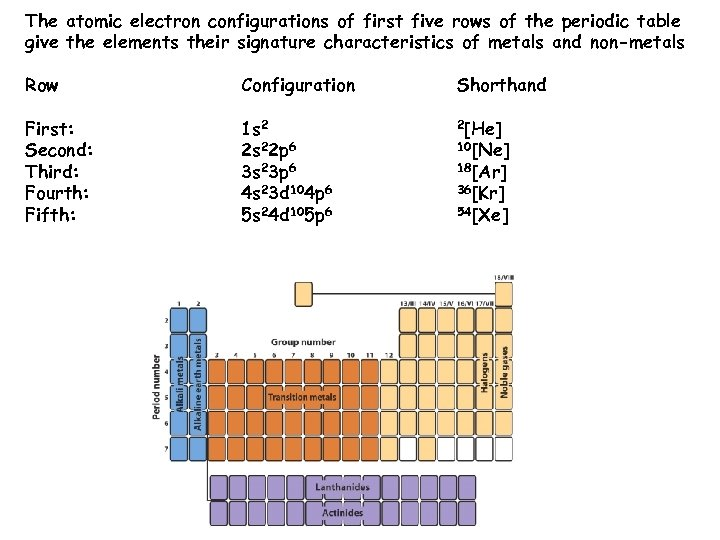The atomic electron configurations of first five rows of the periodic table give the elements their signature characteristics of metals and non-metals Row Configuration Shorthand First: Second: Third: Fourth: Fifth: 1 s 2 2 s 22 p 6 3 s 23 p 6 4 s 23 d 104 p 6 5 s 24 d 105 p 6 2[He] 10[Ne] 18[Ar] 36[Kr] 54[Xe]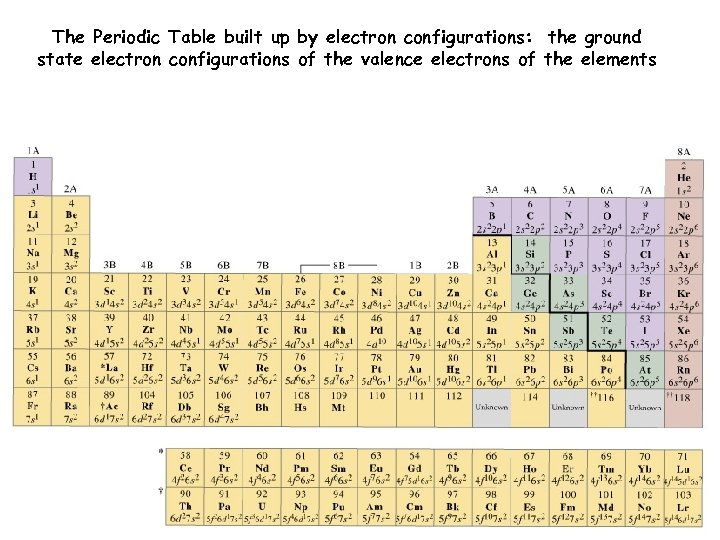The Periodic Table built up by electron configurations: the ground state electron configurations of the valence electrons of the elements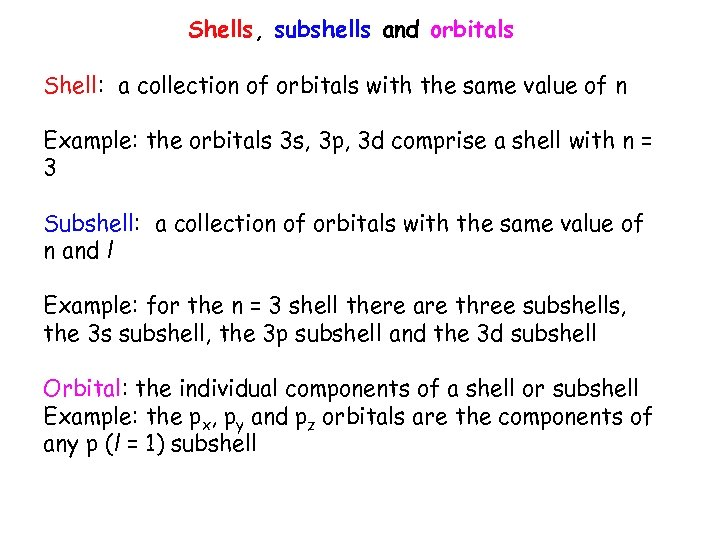Shells, subshells and orbitals Shell: a collection of orbitals with the same value of n Example: the orbitals 3 s, 3 p, 3 d comprise a shell with n = 3 Subshell: a collection of orbitals with the same value of n and l Example: for the n = 3 shell there are three subshells, the 3 s subshell, the 3 p subshell and the 3 d subshell Orbital: the individual components of a shell or subshell Example: the px, py and pz orbitals are the components of any p (l = 1) subshell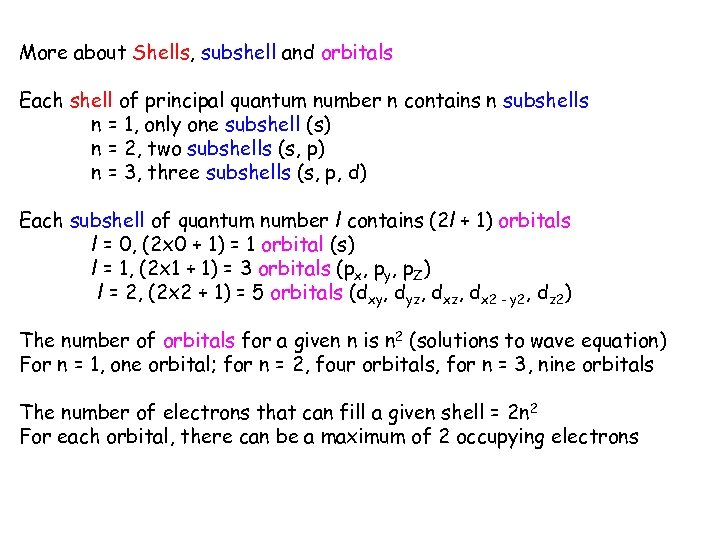More about Shells, subshell and orbitals Each shell of principal quantum number n contains n subshells n = 1, only one subshell (s) n = 2, two subshells (s, p) n = 3, three subshells (s, p, d) Each subshell of quantum number l contains (2 l + 1) orbitals l = 0, (2 x 0 + 1) = 1 orbital (s) l = 1, (2 x 1 + 1) = 3 orbitals (px, py, p. Z) l = 2, (2 x 2 + 1) = 5 orbitals (dxy, dyz, dx 2 - y 2, dz 2) The number of orbitals for a given n is n 2 (solutions to wave equation) For n = 1, one orbital; for n = 2, four orbitals, for n = 3, nine orbitals The number of electrons that can fill a given shell = 2 n 2 For each orbital, there can be a maximum of 2 occupying electrons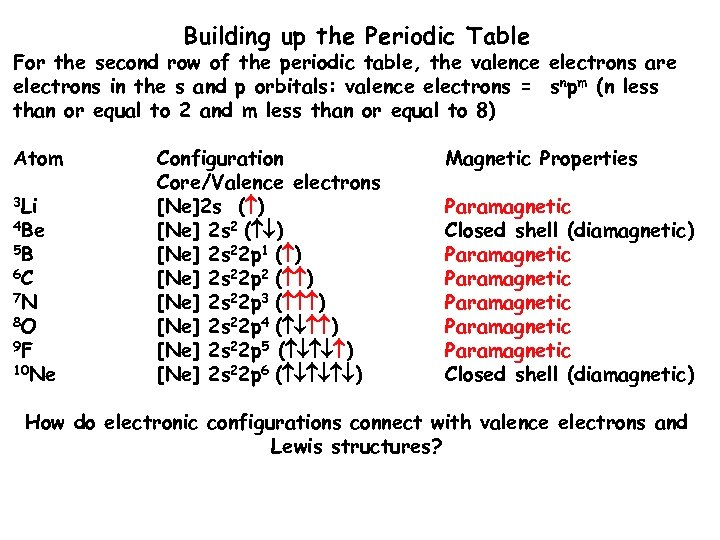Building up the Periodic Table For the second row of the periodic table, the valence electrons are electrons in the s and p orbitals: valence electrons = snpm (n less than or equal to 2 and m less than or equal to 8) Atom 3 Li 4 Be 5 B 6 C 7 N 8 O 9 F 10 Ne Configuration Core/Valence electrons [Ne]2 s ( ) [Ne] 2 s 22 p 1 ( ) [Ne] 2 s 22 p 2 ( ) [Ne] 2 s 22 p 3 ( ) [Ne] 2 s 22 p 4 ( ) [Ne] 2 s 22 p 5 ( ) [Ne] 2 s 22 p 6 ( ) Magnetic Properties Paramagnetic Closed shell (diamagnetic) Paramagnetic Paramagnetic Closed shell (diamagnetic) How do electronic configurations connect with valence electrons and Lewis structures?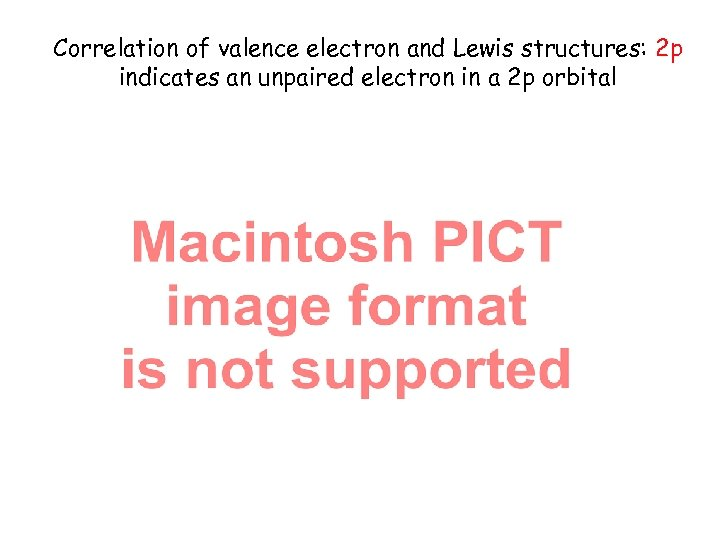Correlation of valence electron and Lewis structures: 2 p indicates an unpaired electron in a 2 p orbital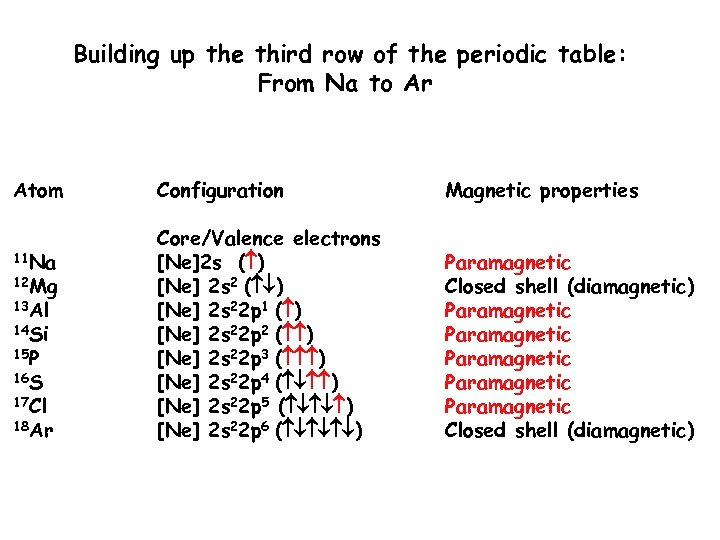Building up the third row of the periodic table: From Na to Ar Atom 11 Na 12 Mg 13 Al 14 Si 15 P 16 S 17 Cl 18 Ar Configuration Magnetic properties Core/Valence electrons [Ne]2 s ( ) [Ne] 2 s 22 p 1 ( ) [Ne] 2 s 22 p 2 ( ) [Ne] 2 s 22 p 3 ( ) [Ne] 2 s 22 p 4 ( ) [Ne] 2 s 22 p 5 ( ) [Ne] 2 s 22 p 6 ( ) Paramagnetic Closed shell (diamagnetic) Paramagnetic Paramagnetic Closed shell (diamagnetic)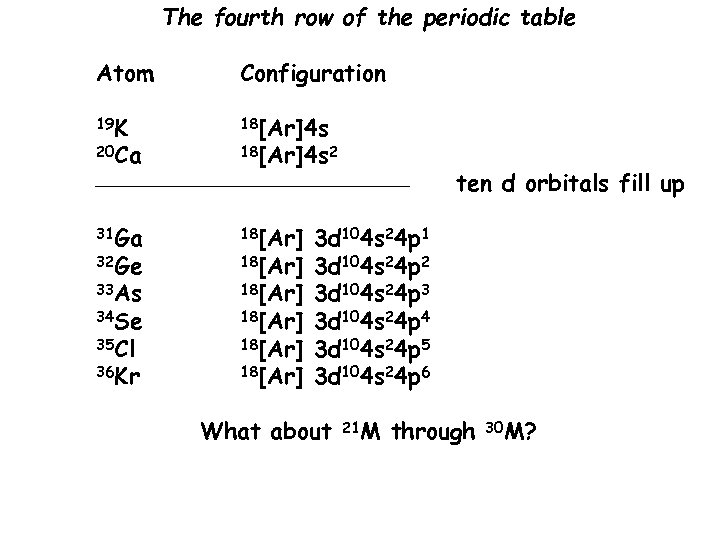The fourth row of the periodic table Atom Configuration 19 K 18[Ar]4 s 20 Ca 18[Ar]4 s 2 _________________ 31 Ga 18[Ar] 33 As 18[Ar] 32 Ge 34 Se 35 Cl 36 Kr 18[Ar] ten d orbitals fill up 3 d 104 s 24 p 1 3 d 104 s 24 p 2 3 d 104 s 24 p 3 3 d 104 s 24 p 4 3 d 104 s 24 p 5 3 d 104 s 24 p 6 What about 21 M through 30 M?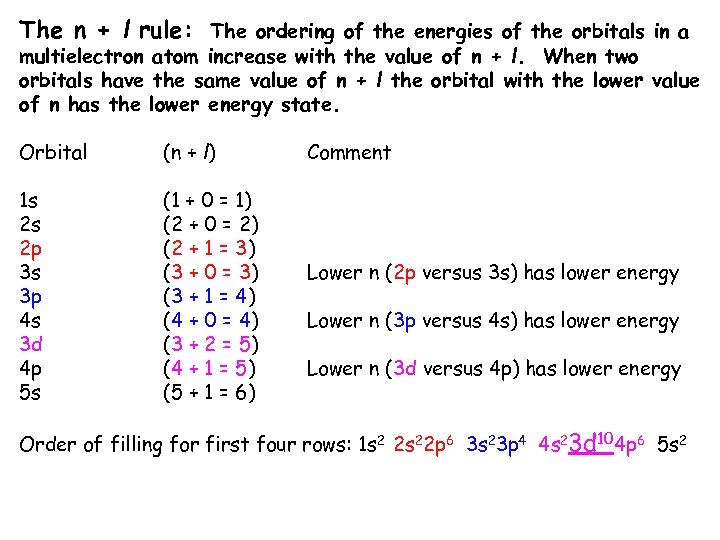The n + l rule: The ordering of the energies of the orbitals in a multielectron atom increase with the value of n + l. When two orbitals have the same value of n + l the orbital with the lower value of n has the lower energy state. Orbital (n + l) 1 s 2 s 2 p 3 s 3 p 4 s 3 d 4 p 5 s (1 + 0 = 1) (2 + 0 = 2) (2 + 1 = 3) (3 + 0 = 3) (3 + 1 = 4) (4 + 0 = 4) (3 + 2 = 5) (4 + 1 = 5) (5 + 1 = 6) Comment Lower n (2 p versus 3 s) has lower energy Lower n (3 p versus 4 s) has lower energy Lower n (3 d versus 4 p) has lower energy Order of filling for first four rows: 1 s 2 2 s 22 p 6 3 s 23 p 4 4 s 23 d 104 p 6 5 s 2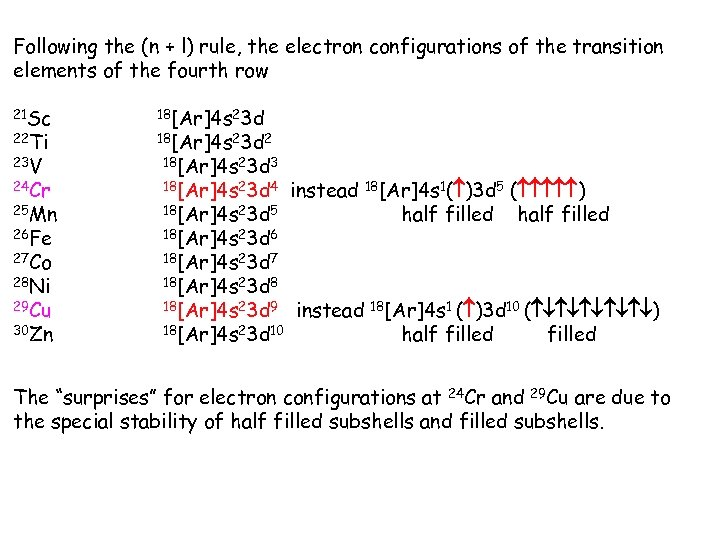Following the (n + l) rule, the electron configurations of the transition elements of the fourth row 21 Sc 22 Ti 23 V 24 Cr 25 Mn 26 Fe 27 Co 28 Ni 29 Cu 30 Zn 18[Ar]4 s 23 d 2 18[Ar]4 s 23 d 3 18[Ar]4 s 23 d 4 18[Ar]4 s 23 d 5 instead 18[Ar]4 s 23 d 6 18[Ar]4 s 1( )3 d 5 ( ) half filled 18[Ar]4 s 23 d 7 18[Ar]4 s 23 d 8 18[Ar]4 s 23 d 9 18[Ar]4 s 23 d 10 instead 18[Ar]4 s 1 ( )3 d 10 ( ) half filled The “surprises” for electron configurations at 24 Cr and 29 Cu are due to the special stability of half filled subshells and filled subshells.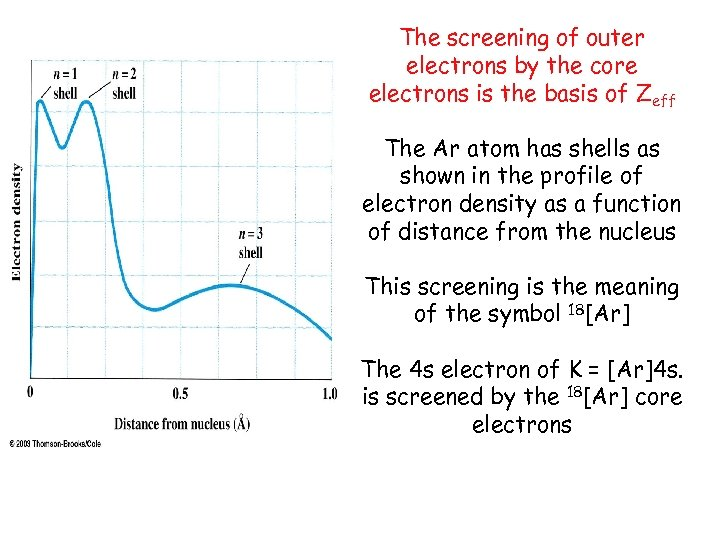The screening of outer electrons by the core electrons is the basis of Zeff The Ar atom has shells as shown in the profile of electron density as a function of distance from the nucleus This screening is the meaning of the symbol 18[Ar] The 4 s electron of K = [Ar]4 s. is screened by the 18[Ar] core electrons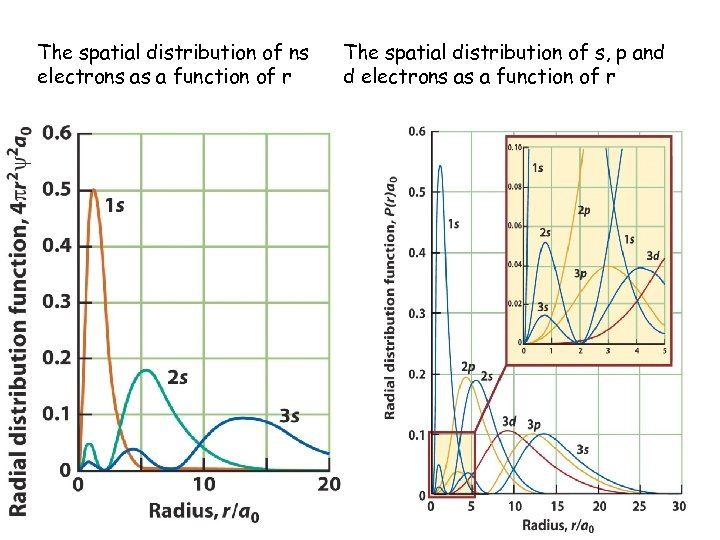The spatial distribution of ns electrons as a function of r The spatial distribution of s, p and d electrons as a function of r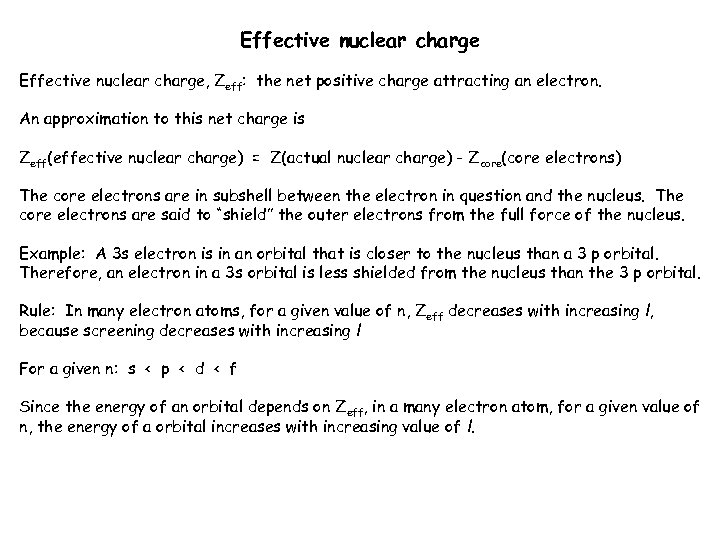Effective nuclear charge, Zeff: the net positive charge attracting an electron. An approximation to this net charge is Zeff(effective nuclear charge) = Z(actual nuclear charge) - Zcore(core electrons) The core electrons are in subshell between the electron in question and the nucleus. The core electrons are said to “shield” the outer electrons from the full force of the nucleus. Example: A 3 s electron is in an orbital that is closer to the nucleus than a 3 p orbital. Therefore, an electron in a 3 s orbital is less shielded from the nucleus than the 3 p orbital. Rule: In many electron atoms, for a given value of n, Zeff decreases with increasing l, because screening decreases with increasing l For a given n: s < p < d < f Since the energy of an orbital depends on Zeff, in a many electron atom, for a given value of n, the energy of a orbital increases with increasing value of l.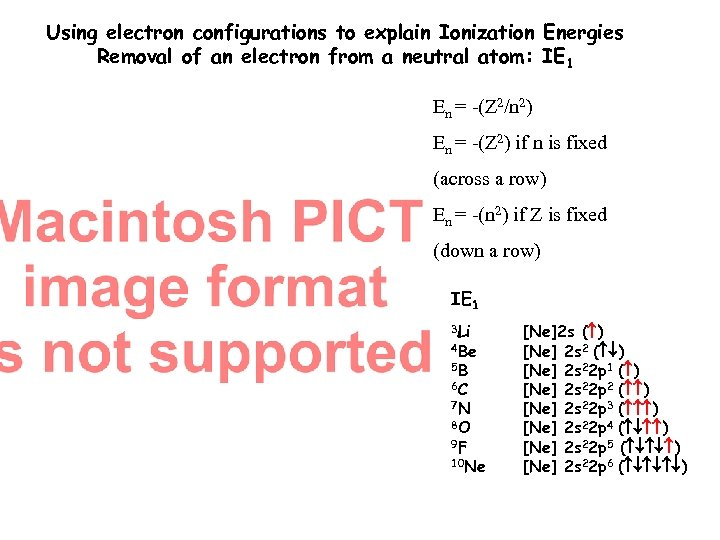Using electron configurations to explain Ionization Energies Removal of an electron from a neutral atom: IE 1 En = -(Z 2/n 2) En = -(Z 2) if n is fixed (across a row) En = -(n 2) if Z is fixed (down a row) IE 1 3 Li 4 Be 5 B 6 C 7 N 8 O 9 F 10 Ne [Ne]2 s ( ) [Ne] 2 s 22 p 1 ( ) [Ne] 2 s 22 p 2 ( ) [Ne] 2 s 22 p 3 ( ) [Ne] 2 s 22 p 4 ( ) [Ne] 2 s 22 p 5 ( ) [Ne] 2 s 22 p 6 ( )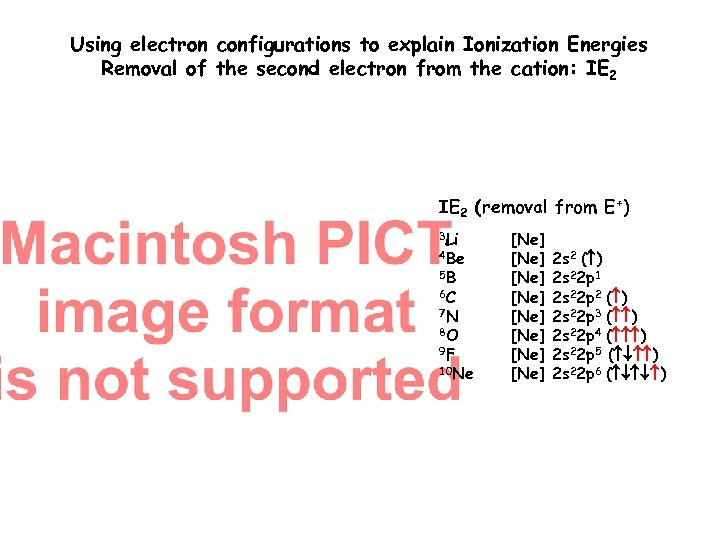Using electron configurations to explain Ionization Energies Removal of the second electron from the cation: IE 2 (removal from E+) 3 Li 4 Be 5 B 6 C 7 N 8 O 9 F 10 Ne [Ne] [Ne] 2 s 2 ( ) 2 s 22 p 1 2 s 22 p 2 ( ) 2 s 22 p 3 ( ) 2 s 22 p 4 ( ) 2 s 22 p 5 ( ) 2 s 22 p 6 ( )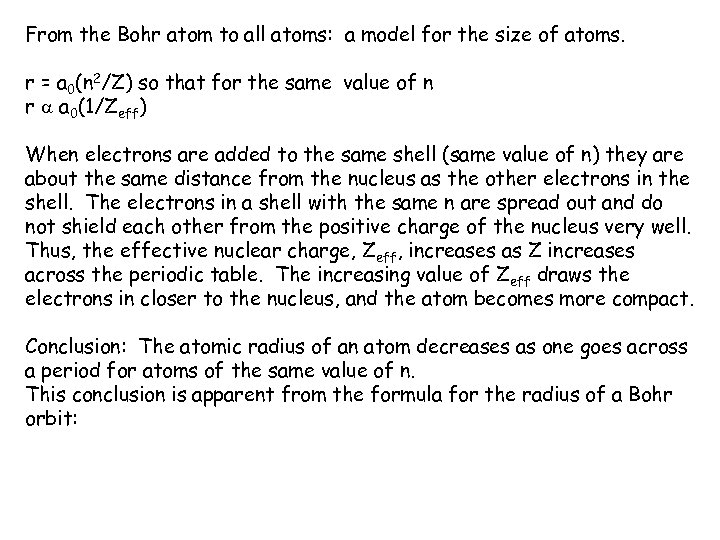From the Bohr atom to all atoms: a model for the size of atoms. r = a 0(n 2/Z) so that for the same value of n r a a 0(1/Zeff) When electrons are added to the same shell (same value of n) they are about the same distance from the nucleus as the other electrons in the shell. The electrons in a shell with the same n are spread out and do not shield each other from the positive charge of the nucleus very well. Thus, the effective nuclear charge, Zeff, increases as Z increases across the periodic table. The increasing value of Zeff draws the electrons in closer to the nucleus, and the atom becomes more compact. Conclusion: The atomic radius of an atom decreases as one goes across a period for atoms of the same value of n. This conclusion is apparent from the formula for the radius of a Bohr orbit: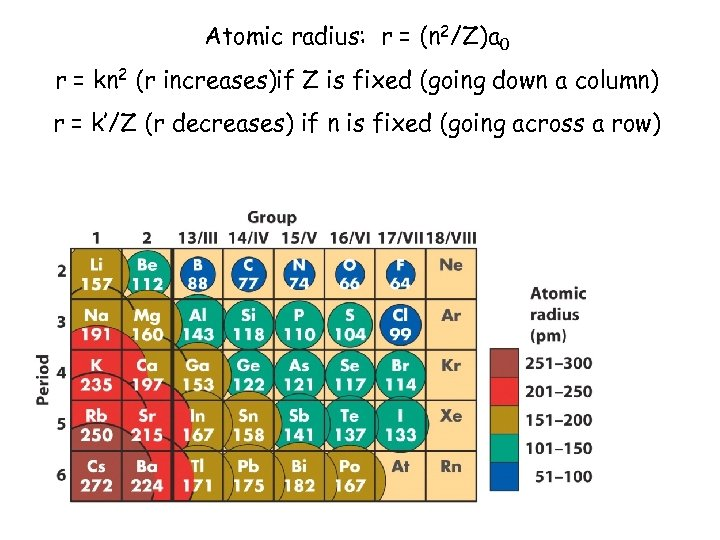Atomic radius: r = (n 2/Z)a 0 r = kn 2 (r increases)if Z is fixed (going down a column) r = k’/Z (r decreases) if n is fixed (going across a row)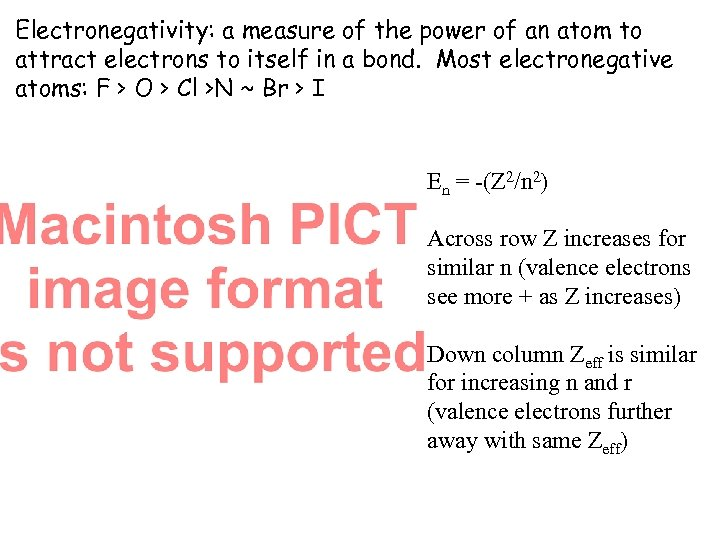Electronegativity: a measure of the power of an atom to attract electrons to itself in a bond. Most electronegative atoms: F > O > Cl >N ~ Br > I En = -(Z 2/n 2) Across row Z increases for similar n (valence electrons see more + as Z increases) Down column Zeff is similar for increasing n and r (valence electrons further away with same Zeff)